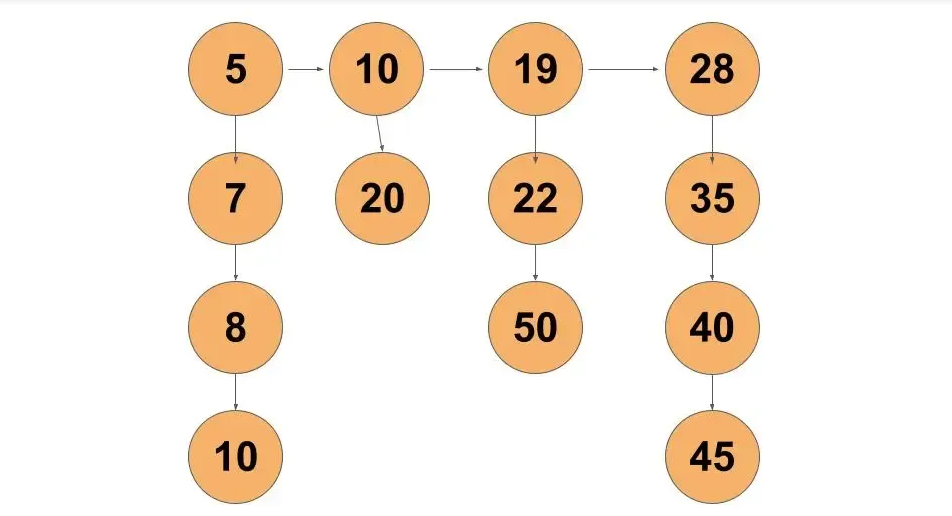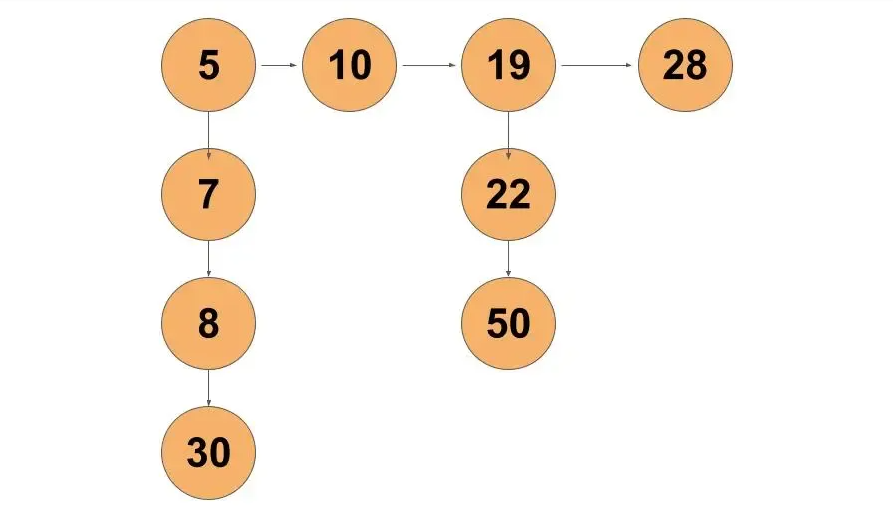Problem Statement: Given a Linked List of size N, where every node represents a sub-linked-list and contains two pointers:

(i) a next pointer to the next node,

(ii) a bottom pointer to a linked list where this node is head.

Each of the sub-linked-list is in sorted order.

Flatten the Link List such that all the nodes appear in a single level while maintaining the sorted order.

Note: The flattened list will be printed using the bottom pointer instead of the next pointer.

Examples:

```Example 1:
Input:
Number of head nodes = 4
Array holding length of each list with head and bottom = [4,2,3,4]
Elements of entire linked list = [5,7,8,30,10,20,19,22,50,28,35,40,45]Output:
Flattened list = [5,7,8,10,19,20,22,28,30,35,40,45,50]
Explanation:
Flattened list is the linked list consisting entire elements of the given list in sorted order```
```Example 2:
Input:
Number of head nodes = 4
Array holding length of each list with head and bottom = [4,1,3,1]
Elements of entire linked list = [5,7,8,30,10,19,22,50,28]Output:
Flattened list = [5,7,8,10,19,22,28,30,50]
Explanation:
Flattened list is the linked list consisting entire elements of the given list in sorted order
```

Solution:

Approach:

Since each list, followed by the bottom pointer, are in sorted order. Our main aim is to make a single list in sorted order of all nodes. So, we can think of a merge algorithm of merge sort.

The process to flatten the given linked list is as follows:-

• We will recurse until the head pointer moves null. The main motive is to merge each list from the last.
• Merge each list chosen using the merge algorithm. The steps are
• Create a dummy node. Point two pointers, i.e, temp and res on dummy node. res is to keep track of dummy node and temp pointer is to move ahead as we build the flattened list.
• We iterate through the two chosen. Move head from any of the chosen lists ahead whose current pointed node is smaller.
• Return the new flattened list found.

Dry Run:

We will assign individual lists with bottom pointers names as l1, l2, l3, and l4 respectively for our convenience.

Let’s see the recursion tree of the function flatten and merge function. It will trace down to allow the merge function to merge two sorted lists from the end.

The merge function works like the merge algorithm of merge sort. Firstly, the algorithm will merge l3,l4 lists.

Now, pointer b is null. So, we will merge the remaining nodes of pointer a until node a reaches null.

The same way other pairs of lists will be merged.

Code:

## C++ Code

``````Node* mergeTwoLists(Node* a, Node* b) {

Node *temp = new Node(0);
Node *res = temp;

while(a != NULL && b != NULL) {
if(a->data < b->data) {
temp->bottom = a;
temp = temp->bottom;
a = a->bottom;
}
else {
temp->bottom = b;
temp = temp->bottom;
b = b->bottom;
}
}

if(a) temp->bottom = a;
else temp->bottom = b;

return res -> bottom;

}
Node *flatten(Node *root)
{

if (root == NULL || root->next == NULL)
return root;

// recur for list on right
root->next = flatten(root->next);

// now merge
root = mergeTwoLists(root, root->next);

// return the root
// it will be in turn merged with its left
return root;
}``````

Time Complexity: O(N), where N is the total number of nodes present

Reason: We are visiting all the nodes present in the given list.

Space Complexity: O(1)

Reason: We are not creating new nodes or using any other data structure.

## Java Code

``````class TUF
{
Node mergeTwoLists(Node a, Node b) {

Node temp = new Node(0);
Node res = temp;

while(a != null && b != null) {
if(a.data < b.data) {
temp.bottom = a;
temp = temp.bottom;
a = a.bottom;
}
else {
temp.bottom = b;
temp = temp.bottom;
b = b.bottom;
}
}

if(a != null) temp.bottom = a;
else temp.bottom = b;
return res.bottom;

}
Node flatten(Node root)
{

if (root == null || root.next == null)
return root;

// recur for list on right
root.next = flatten(root.next);

// now merge
root = mergeTwoLists(root, root.next);

// return the root
// it will be in turn merged with its left
return root;
}
}``````

Time Complexity: O(N), where N is the total number of nodes present

Reason: We are visiting all the nodes present in the given list.

Space Complexity: O(1)

Reason: We are not creating new nodes or using any other data structure.

## Python Code

``````class Node:
def __init__(self, val):
self.val = val
self.next = None
self.bottom = None

def mergeTwoLists(a, b):
temp = Node(0)
res = temp
while a != None and b != None:
if a.val < b.val:
temp.bottom = a
temp = temp.bottom
a = a.bottom
else:
temp.bottom = b
temp = temp.bottom
b = b.bottom
if a:
temp.bottom = a
else:
temp.bottom = b
return res.bottom

def flatten(root):
if root == None or root.next == None:
return root
# recur for list on right
root.next = flatten(root.next)

# now merge
root = mergeTwoLists(root, root.next)

# return the root
# it will be in turn merged with its left
return root``````

Time Complexity: O(N), where N is the total number of nodes present

Reason: We are visiting all the nodes present in the given list.

Space Complexity: O(1)

Reason: We are not creating new nodes or using any other data structure.